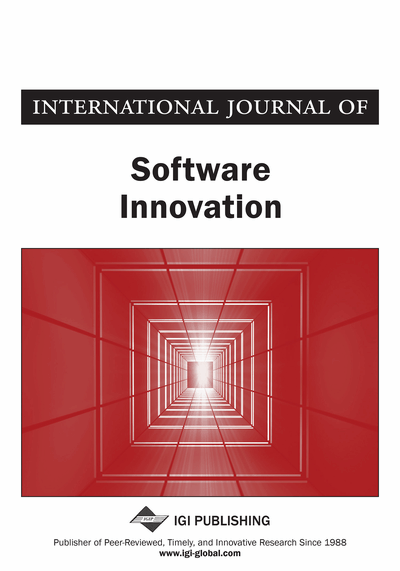# Parallel Online Exact Summation of Floating-point Numbers by Applying MapReduce of Java8

Naoshi Sakamoto (Tokyo Denki University, Tokyo, Japan)
Copyright: © 2017 |Pages: 16
DOI: 10.4018/IJSI.2017040102
OnDemand PDF Download:
Available
\$37.50
No Current Special Offers

## Abstract

Java8 introduced the notion of streams that is a new data structure and supports multi-core processors. When the sum method is called for a stream of floating-point numbers, the summation is calculated at high-speed by applying MapReduce, which distributes computations to cores. However, since floating-point calculation causes an error, simple adaptation of this method cannot determine the result uniquely. Then, in this study, the authors develop a summation program that can be applied to a stream with MapReduce. Their method can calculate at high-speed with keeping correctly rounded.
Article Preview

### 1. INTRODUCTION

Java8 introduces the notion of streams, a new data structure. This is for high-speed calculation technology brought by introducing MapReduce, which applies to multi-core processors, and FunctionalInterface, a new syntax.

FunctionalInterface is an extension of the former anonymous class. An interface is called FunctionalInterface when it only contains a single abstract method. A “lambda formula”, a new syntax, is a literal of a FunctionalInterface. This is corresponding to an instance of the class implemented a single method to an anonymous class. By this extension, while methods are not still the first class object in Java, we can deal with the object of FunctionalInterface as a function object (Figure 1, l.5).

For a set of data and a given function, a Map process generates a set of results by applying the function for each data (Figure 1, ll. 5–7). By assuming that the function has no adverse reaction, each calculation of the function can be distributed to cores. Note that the processing order to the data is not determined uniquely.

On the other hand, for a set of data and a given binary operator, a Reduce process calculates aggregation for the operator by parallel processing (Figure 1, ll. 9–11). This is not so executed that each data and the intermediate result are binary-operated, and the result is let to be the new intermediate result one by one. But, this is so executed as making each data correspond to a leaf of a complete binary tree, then binary-operates at each position of medium nodes.

It has been well-known that summation for floating-point numbers causes a rounding error. It is also known that adding numbers in order from the smallest number to the largest number reduces the rounding error. That is, the order of adding for floating-point numbers affects the results. Thus, adding floating-point numbers does not satisfy the associative law.

Nevertheless, adding floating-point numbers used to be executed with paying less attention to the error. Fortunately, since formerly adding has been executed in order, the results are the same for any conditions of any computers. On the other hand, the sum method of java.util.stream.DoubleStream of Java8 is also implemented with paying less attention to the error. However, by introducing the notion of stream, Reduce causes the discrepancy of the summation result. That is, the result of sum method of java.util.stream.DoubleStream may cause the discrepancy of the result for the environment and the condition of computers.

On the other hand, Java is equipped with BigDecimal for arbitrary-precision arithmetic. Then, we can calculate without errors by applying BigDecimal to stream (Figure 2). However, in spite of the benefits of multi-core, it spends quite long time.

In this study, we develop a stable fast program for summation of floating-point numbers by using Stream. The proposal program is independent from the order of numbers, has the same precision as BigDecimal, is at most three times slower than sum method of DoubleStream. Since basic technique called AddTwo contains six additions, the measured running time is seemed be quite good.

This paper is organized as follows: Section 2 gives basic notions, and introduces AddTwo, iFastSum, and Online Exact Sum as the essential basic notions. Section 3 explains the proposal program. Section 4 shows the result of experiments, and evaluation. Finally, Section 5 concludes.

## Complete Article List

Search this Journal:
Reset
Open Access Articles
Volume 10: 4 Issues (2022): Forthcoming, Available for Pre-Order
Volume 9: 4 Issues (2021): 3 Released, 1 Forthcoming
Volume 8: 4 Issues (2020)
Volume 7: 4 Issues (2019)
Volume 6: 4 Issues (2018)
Volume 5: 4 Issues (2017)
Volume 4: 4 Issues (2016)
Volume 3: 4 Issues (2015)
Volume 2: 4 Issues (2014)
Volume 1: 4 Issues (2013)
View Complete Journal Contents Listing